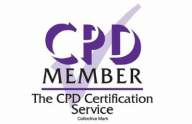• No products in the cart.

### Course Curriculum

 Integers ( Directed Numbers) Lecture 1 Introduction 00:02:00 Lecture 2 Quick glance at number system 00:05:00 Lecture 3 Integers and ordering of integers 00:06:00 Lecture 4 Addition and Subtractions of Integers 00:07:00 Lecture 5 Operations on Integers 00:04:00 Lecture 6 Multiplication and Division of Integers 00:04:00 Lecture 7 Powers of Integers (Exponents) 00:04:00 Factors and Multiples Lecture 8 Complete knowledge about factors and multiples 00:06:00 Lecture 9 Divisibility tests for 2,3,4,5,6 00:07:00 Lecture 10 Divisibility rules 7,8,9,10,11 00:06:00 Lecture 11 Prime Factorization 00:06:00 Lecture 12 Highest Common Factor HCF 00:10:00 Lecture 13 Least Common Multiple LCM 00:16:00 Lecture 14 Relation between LCM and HCF 00:04:00 Fractions Lecture 15 Classification of Fractions 00:04:00 Lecture 16 Convert mixed to improper and improper to mixed fractions 00:03:00 Lecture 17 Equivalent Fractions 00:04:00 Lecture 18 Comparing Fractions after converting to like fractions 00:09:00 Lecture 19 Reducing a fraction to lowest term 00:04:00 Lecture 20 Addition and subtraction OF fractions 00:08:00 Lecture 21 Multiplication and division of fractions 00:11:00 Lecture 22 Word problems on fractions 00:16:00 Simplification Rule : BODMAS Lecture 23 BODMAS and rules to remove brackets 00:03:00 Lecture 24 Simplify expressions using BODMAS rules 00:05:00 Lecture 25 Simplify expressions involving fractions using BODMAS 00:07:00 Decimal numbers Lecture 26 Decimal numbers in relation to fractions 00:08:00 Lecture 27 Like and unlike Decimals 00:05:00 Lecture 28 Ordering of Decimal numbers 00:10:00 Lecture 29 Addition and subtraction of Decimal 00:07:00 Lecture 30 Multiplication of Decimal 00:03:00 Lecture 31 Division of Decimal 00:05:00 Rational Numbers Lecture 32 Rational number 00:02:00 Lecture 33 Representation of Rational Numbers on Number line 00:04:00 Lecture 34 Determining which fraction is terminating or non terminating 00:05:00 Lecture 35 shortcut method of writing decimal expansion of fraction to decimal 00:08:00 Approximation Lecture 36 Rounding to whole number 00:06:00 Lecture 37 Rounding to required number of decimals (1 d.p. ) 00:05:00 Lecture 38 rounding to significant digits 00:07:00 Lecture 39 Practice question on three methods of approximation 00:05:00 Estimation and Scientific Notation or Standard form Lecture 40 Estimation 00:05:00 Lecture 41 Scientific Notation 00:07:00 Lecture 42 Operation on numbers when they are in scientific notation 00:06:00 Percentage Lecture 43 Percentage to fraction and fraction to percentage 00:06:00 Lecture 44 Percentage of a quantity and conversation to decimal 00:06:00 Lecture 45 Expressing one quantity as percentage of another quantity 00:06:00 Lecture 46 Finding increase decrease percent 00:05:00 Lecture 47 Uses of percentages-Word Problems 00:09:00 Ratio and Proportion Lecture 48 Ratio- How to express as simple ratio 00:09:00 Lecture 49 How to compare Ratios 00:04:00 Lecture 50 Word problems on finding ratios 00:07:00 Lecture 51 To divide a given quantity into a given Ratio 00:11:00 Lecture 52 Proportion 00:04:00 Lecture 53 Practice problems on Proportion 00:06:00 Lecture 54 Continued proportion 00:06:00 Unitary Method and its Applications Lecture 55 Direct Variation ( or proportion) 00:04:00 Lecture 56 Problems based on Direct proportion 00:10:00 Lecture 57 Inverse Variation ( or proportion) 00:10:00 Lecture 58 Multiple Ratios 00:10:00 Profit , Loss, discount and Tax Lecture 59 Basics – Profit and Loss 00:04:00 Lecture 60 More practice problems on Profit & Loss 00:06:00 Lecture 61 Selling price formula and Problems 00:04:00 Lecture 62 Cost price formula and Problems 00:03:00 Lecture 63 Higher problems on Profit and Loss 00:08:00 Lecture 64 Basics – Discount.mp4 00:05:00 Lecture 65 Practice problems on Discount 00:04:00 Lecture 66 Tax 00:06:00 Mock Exam Mock Exam – Mathematics for Beginners 00:20:00 Final Exam Final Exam – Mathematics for Beginners 00:20:00 Order Your Certificate Order Your Certificate New 00:00:00

# Mathematics for Beginners

4.7( 8 REVIEWS )
288 STUDENTS
\$346.11• Learning outcome• Learn do calculations faster in an easier way​
• Brush up Your basics of Middle school and high school Mathematics.​
• Solve word problems on profit, loss, percentage and tax with comfort​
• Solve real life-based problems with fractions, decimals, ratios, and estimations with ease​• Requirements
• Anyone from any academic background can take this course.
• Why should I take this course?
• 7.5+ hours of high-quality e-learning content. ​
• Course completion certificate.
• Internationally recognized accredited qualification.
• Revise all kinds of fundamental mathematical problems before sitting for your SAT exams.
• Brush up Your basics of Middle school and high school Mathematics.Testimonial

Hear what our happy students want to say